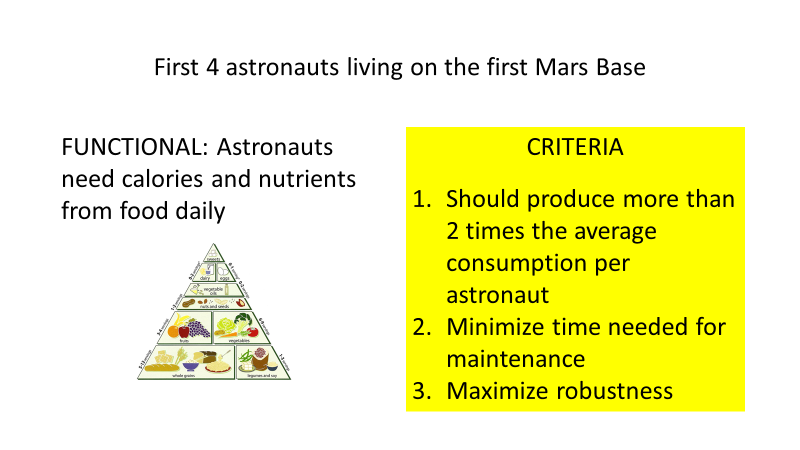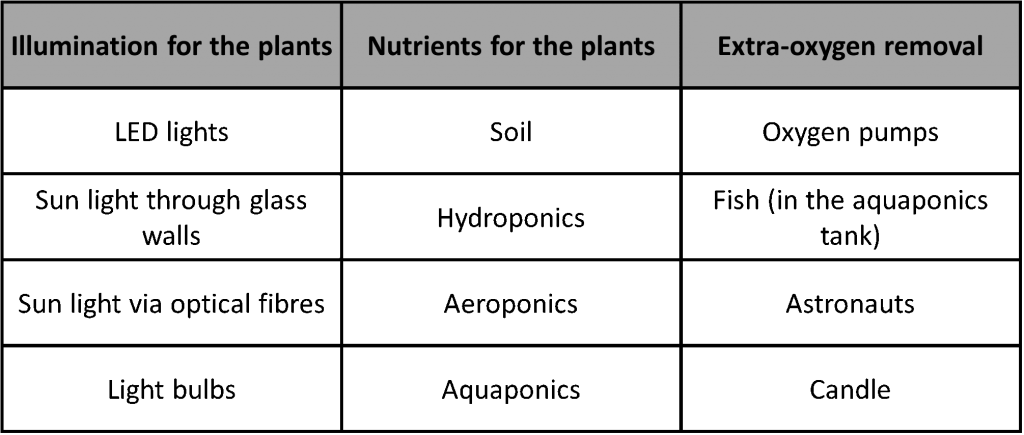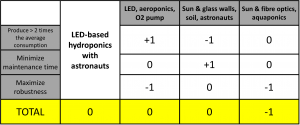Categories

# Exercise: Problem definition, morphological table and decision-making matrix

by Heikki Immonen

By doing this exercise you will get familiar at using three tools that make solving complex and big problems easier and more effective.
The output of this exercise is a presentation with 13 slides:

1. Title
2. Hook
3. Team introduction
4. Problem introduction
5. Main stakeholder problem definition
6. Problem structure
7. Morphological table
8. Concept 1
9. Concept 2
10. Concept 3
11. Reference concept
12. Decision-making matrix
13. Final concept

## 1. Title

This slide contains the title of your presentation.

For example:

Mars Greenhouse Concept

New Student Café.

## 2. Hook

Share something unexpected and interesting related to the problem you are solving.

For example:

Did you that there are growing techniques that are 100 times more efficient than traditional open air agriculture?

## 3. Team introduction

Who are the people in your team and what aspects of your background gives you authority on the topic of your presentation?

## 4. Problem introduction

General introduction of the problem you are trying to solve or the solution you are trying to develop.

For example:

We want to develop a food growing system for Mars

Our plan is to create a new café for students on campus.

## 5. Main stakeholder problem definition

Show the problem definition written from the perspective of your main stakeholder aka. the customer. The problem definition must include three elements Customer segment, Customer Problem, Customer Criteria.

Customer problem should be written based on the main type of need the your solution should satisfy for your customer: functional, emotional, social.

List of customer criteria should have 3 – 4 criteria your customer chooses when he/she compares different solutions.

For example:## 6. Problem structure

Explain how you have divided the problem in to smaller problems. Ideal number of sub-problems would be 3 – 5.

For example:

1. Providing illumination for plants, 2. providing nutrients for the plants and 3. how to get rid of the oxygen produced by the plants are three important sub-problems of our challenge.

1. Menu, 2. Location, and 3. Interior design are the main sub-problems when you are planning for a new café.

## 7. Morphological table

Show the morphological table of your problem with 3 – 5 sub-problems (columns) and 3 – 5 ideas per sub-problem (rows under the top row).

For example:## 8. Concept 1

Introduce here your first concept that you generate using the morphological table. The concept should be a combination of at least one idea from each column of your morphological table. This and the other concepts should be as different from each other as possible.

For example:

Our first concept is based on the following combination: LED lights + Aeroponics + Candle

## 9. Concept 2

Introduce here your second concept that you generate using the morphological table. The concept should be a combination of at least one idea from each column of your morphological table. This and the other concepts should be as different from each other as possible.

## 10. Concept 3

Introduce here your third concept that you generate using the morphological table. The concept should be a combination of at least one idea from each column of your morphological table. This and the other concepts should be as different from each other as possible.

## 11. Reference concept

Introduce here your reference concept that you will use for evaluating your own concepts. The reference concept should be an already existing solution that is popular among your customer segment.

For example:

Our reference concept is the small Veggie growing system developed by NASA and which is operational at the international space station (ISS). It’s based on LED’s + hydroponics + astronauts.

## 12. Decision-making matrix

Show here your decision-making matrix with evaluation scores and calculated total points.

For example:## 13. Final concept

Introduce here your final concept that you generated based on the evaluations and by combining the best feature in to a one best solution

For example:

Our final best concept is based on the following combination: LED lights + Aeroponics + astronauts